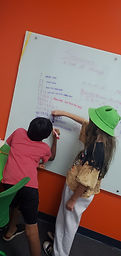Mr. Jake

Target 1​

Lesson Type:

Review

Algebra

:

Sequence

Use arithmetic to define a sequence and calculate the next unit in a series.

1:

Write a pattern based on a given rule.

2:

Write an arithmetic rule to explain a given sequence.

3:

Understand that a pattern can be described by stating the rule that it is following.

4:

Define multiplication and division patterns.

6th

Vocabulary:

Sequences, Rule, Pattern

Activities:

Determined the rule for given sequencesHome Exploration

Guiding Questions:Absent Students:

Target 2

:

1:

Understand equivalent measurements.

2:

Understand how to convert from one unit to another.

3:

Use scale to reproduce a figure at either a bigger or smaller size (relate the measurement of the original figure to the new figure).

6th

Vocabulary:

Unit, conversion, Rate

Activities:

Explained the different types of measurment that can be quantified

Converted between units give conversion rateHome Exploration

Guiding Questions:Target 3

:

Vocabulary:

Activities:Home Exploration